# Sizeof and Limits of Data Type in C

## Sizeof and Limits of Data Type in C Language

In this article, I am going to discuss Sizeof and Limits of Data Types in C Language with Examples. In our last article, we discussed Character Data Type in C Language briefly. In this article, we are going to discuss two more concepts related to data type. The first one is the sizeof method or we can also call it sizeof operator and the second one is the limits of each data type.

##### Sizeof() Fucntion in C Language

The sizeof function is a predefined function just like the printf and scanf() functions.

##### What is the use of sizeof function in C?

The sizeof function in C Language is used to return the size of different things. So, what are the different things? The sizeof function returns the size of the following four things.

1. Size of a variable.
2. Size of a data type
3. Size of an expression
4. Size of a pointer

So, we can pass either variable, data type, expression, or pointer as an argument to the sizeof function. The sizeof function is a predefined function and that will return the size of different types of things. So, if you want to find out the size of any data type, we can go for a sizeof function. For better understanding, please have a look at the following diagram.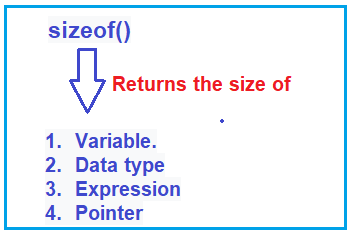##### Predefined sizeof function example in c language:

Please have a look at the following example which uses the predefined sizeof function.

```#include<stdio.h>
#include<conio.h>
int main()
{
char C;
short S;
printf("size of char : %d bytes(s)\n", sizeof(C));
printf("size of short : %d bytes(s)\n", sizeof(S));
printf("size of float : %d bytes(s)\n", sizeof(float));
return 0;
}
```

In the above program, first, I am declaring two variables. One is of type character i.e. C and the second one is of type short i.e. s. Then I print the size of these two variables using the sizeof function.

What is the size? The size representation is always in the form of integers. So always use %d format specifier to show the size. Here, we are passing the variable names to the sizeof function. When we pass C variable name to the sizeof function, then it will return the size of the character. The size of the character is one byte. so it will print that value one.

Next, it will send the control to the next line. In the next line, we are printing the size of short, it is also %d because size is always in integers. And we know the size of the short is 2 bytes, so it will print 2 and send the control to the next line.

Whenever we are the calling sizeof function, we can pass either variable name, data type name, expression or pointer. In the next line, we are passing the data type float to the sizeof function. The size of the float is 4 bytes. So here it will print the value as 4 bytes.

So, when you run the above program, you will get the following output.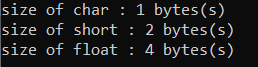In our upcoming articles, we discuss how to pass expression and pointer to the sizeof function in C Language.

##### Limits of data type in C Language

Now we will understand the limits of a data type. What are the limits of a data type means we have one header file i.e. limits.h. The Limits.h header file contains n number of predefined variables and all these predefined variables are global variables. Global variable means we can access these variables from anywhere in any c application. These variables are also called constant variables. Constant variable means we cannot modify the values of these variables. We cannot modify limits.h header file. For better understanding, please have a look at the following diagram.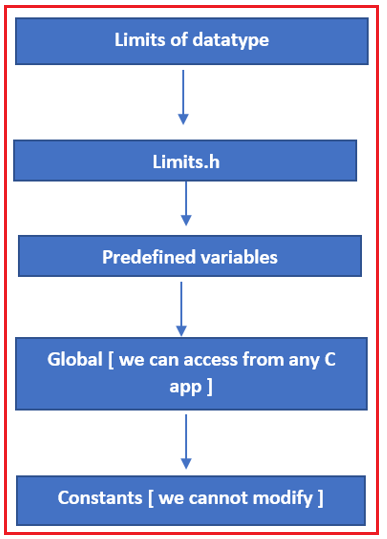Now we will see some programs on how to use a sizeof function and then we will see how to work with the limits.h variables.

##### Example to understand limits of data type in C Language:

Now, we will see how to print the limits of each data type. The Limits.h header file contain so many predefined constant variables i.e global variables. The limits.h header contains many predefined variables as shown in the below image,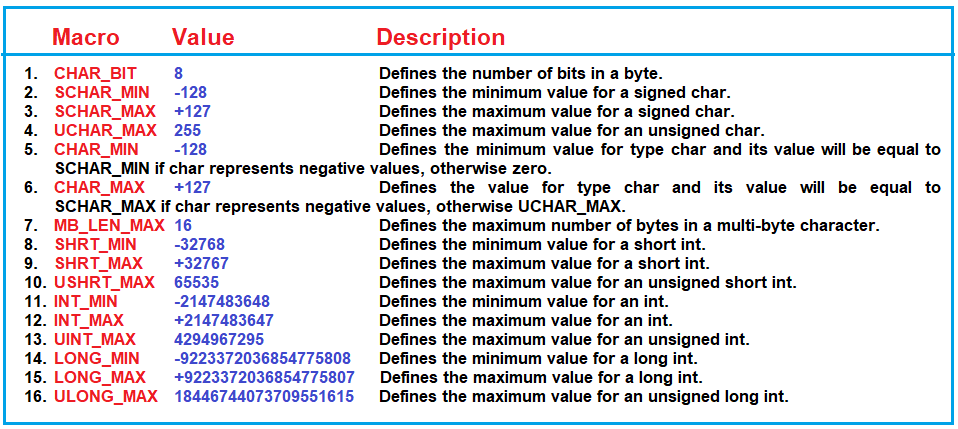In any programming language, if you want to represent a constant variable. Then mostly, we are using capital letters only. All these variables are belonging to signed type and unsigned type.

The minimum range of every unsigned datatype starts with a zero. That is why they didn’t provide that info. All these variables are available in a limits.h, and all these are global variables. you can access anywhere in a C application and all these are constants.

##### Example:

Please have a look at the below program.

```#include<stdio.h>
#include<limits.h>
int main()
{
printf("Signed short MIN Value %d\n", SHRT_MIN);
printf("Signed short Max Value %d\n", SHRT_MAX);
printf("Unsigned short Max Value %d\n", USHRT_MAX);
printf("Signed char MIN Value %d\n", SCHAR_MIN);
printf("Signed char Max Value %d\n", SCHAR_MAX);
printf("Unsigned char Max Value %d\n", UCHAR_MAX);
return 0;
}
```

In the above program, we include the limits.h header file. This is because we are using some of the variables which are related to limits.h header file. Or else you will get an error message. When you run the above program, you will get the following output.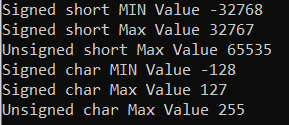Note: You need to remember the limits of each data type if you want to become a good programmer.
In the next article, I am going to discuss Type Casting in C language with Examples. Here, in this article, I try to explain Sizeof and Limits of Data Types in C Language with Examples and I hope you enjoy this Sizeof and Limits of Data Types in the C language article.